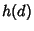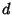## Class Number Formula

A class number formula is a finite series giving exactly the Class Number of a Ring. For a Ring of quadratic integers, the class number is denoted, whereis the discriminant. A class number formula is known for the full ring of cyclotomic integers, as well as for any subring of the cyclotomic integers. This formula includes the quadratic case as well as many cubic and higher-order rings.